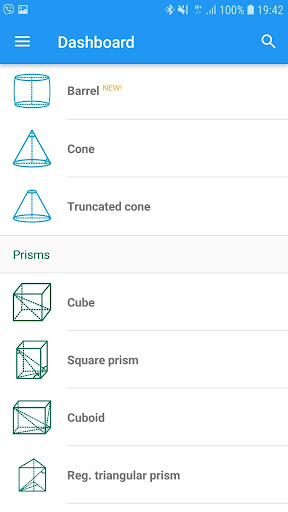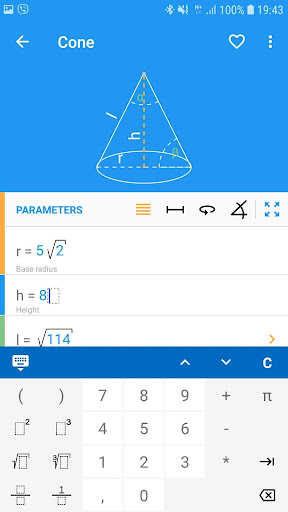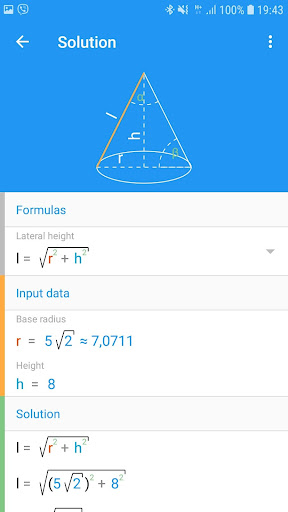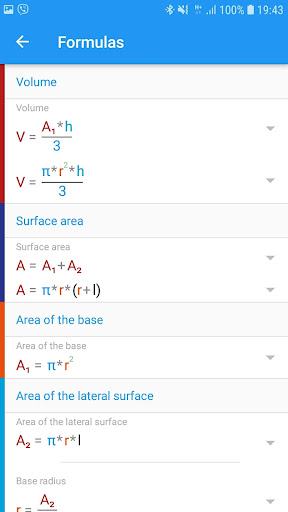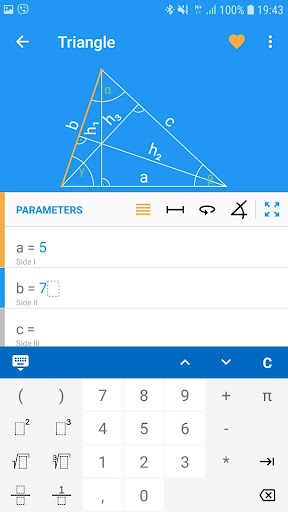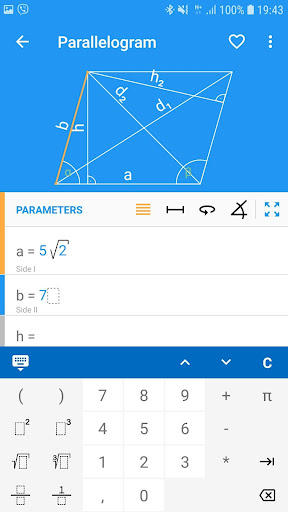#### Geometry PRO v2.12 APK

All you need in geometry. Over 30 figures. Step by step solutions and formulas.
##### Details
Title: Geometry PRO
Genre: Education
Developer: NaNSolvers
##### Description

Geometry PRO is advanced application for solving geometry problems. There is a complete solution delivered for each issue to satisfy every teacher or student.

The application solves every algebraic problem including those with:
– fractions
– roots
– powers
you can also use parentheses, decimal numbers and Pi number.

This application is able to do calculation on the following figures:
– square
– rectangle
– rhombus
– parallelogram
– triangle
– equilateral triangle
– right triangle
– isosceles triangle
– triangle 30-60-90
– circle
– annulus
– trapezoid
– right trapezoid
– isosceles trapezoid
– Pythagorean theorem
– regular hexagon
– sphere
– cylinder
– cone
– regular tetrahedron
– cube
– square prism
– cuboid
– ellipse
– regular Pentagon
– Kite
– trigonometry
– spherical sector
– spherical cap
– annulus sector

PRO version:
– Square pyramid
– Triangular pyramid
– Triangular prism
– Regular triangular prism
– Thales' theorem
– Truncated cone
– Regular octagon
– Regular dodecagon
– Hexagonal prism
– Hexagonal pyramid
– Pentagonal prism
– Barrel
– law of sines
– law of cosines
– spherical wedge
– spherical lune
– spherical segment
– spherical zone

An advanced validation of data entry allows you to find the errors quickly and corrects them for you immediately.

Geometry PRO will calculate all the parameters of the figure, if you enter the necessary data. The order of data entry depends on you!

– Do you want to calculate a side of the square? No problem. Geometry PRO will do it for you.
– Do you have an angle and a side of the right triangle? Perfect. Other values can be calculated.

None of your geometry tasks will be a problem now with Geometry PRO. This application has very advanced, powerful and easy – to – use interface.

Additionally, it contains all the useful formulas which you will need to solve geometry tasks. But thats not enough! You do not have to figure it out how did you get a result. This application not only gives you the solution, it also shows you all the formulas which had been used. Pythagorean Theorem, sines and cosines are no longer a problem.